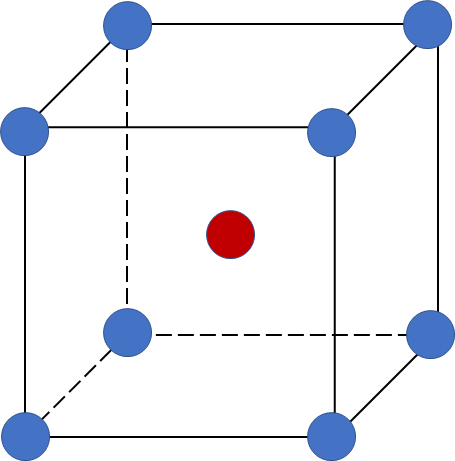# Problem: Lithium crystallizes in a body-centered cubic unit cell. What is the mass of one unit cell in grams?

###### FREE Expert Solution
87% (181 ratings)
###### FREE Expert Solution

We’re being asked to calculate the mass of 1 unit cell when lithium (Li) crystallizes in a body-centered cubic unit cell.

A body-centered cubic (BCC) unit cell is composed of a cube with one atom at each of its corners and one atom at the center of the cubeWe’re going to calculate the mass of 1 unit cell using the following steps:

Step 1: Determine how many atoms are present in 1 unit cell.
Step 2: Calculate how many moles is present in the unit cell.
Step 3: Calculate the mass using the molar mass of the atom.

87% (181 ratings)###### Problem Details

Lithium crystallizes in a body-centered cubic unit cell. What is the mass of one unit cell in grams?

What scientific concept do you need to know in order to solve this problem?

Our tutors have indicated that to solve this problem you will need to apply the Unit Cell concept. You can view video lessons to learn Unit Cell. Or if you need more Unit Cell practice, you can also practice Unit Cell practice problems.

What is the difficulty of this problem?

Our tutors rated the difficulty ofLithium crystallizes in a body-centered cubic unit cell. Wha...as medium difficulty.

How long does this problem take to solve?

Our expert Chemistry tutor, Sabrina took 3 minutes and 27 seconds to solve this problem. You can follow their steps in the video explanation above.

What professor is this problem relevant for?

Based on our data, we think this problem is relevant for Professor Fayissa's class at GMU.